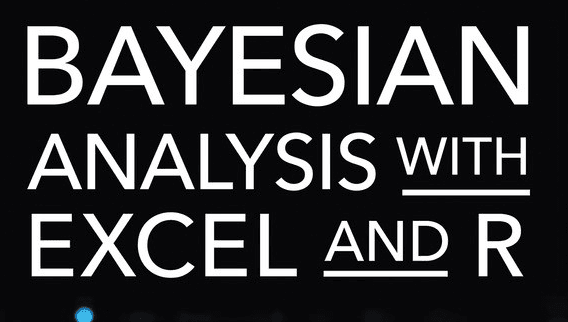# Bayesian Analysis with Excel and R## Bayesian Analysis with Excel and R

Bayesian analysis is a method of statistical inference that uses Bayes’ theorem to update the probability of a hypothesis as more data becomes available. It can be used with both Excel and R, two popular software programs for data analysis.

Excel: Excel is a spreadsheet software that can be used to perform basic Bayesian analysis. Excel has built-in functions that can be used to calculate probability and perform statistical tests. However, Excel is not designed specifically for Bayesian analysis, and it may not have all the features and tools needed for more advanced Bayesian analysis.

R: R is a programming language and software environment for statistical computing and graphics. R is a powerful tool for Bayesian analysis, with a wide variety of packages and libraries available for Bayesian modeling, such as JAGS, Stan, and R-INLA. R also has a large user community, which means that there is a wealth of resources and support available for Bayesian analysis in R.

When using R to do Bayesian Analysis, it’s important to have a good understanding of the Bayesian theory and the R syntax. The packages used for Bayesian analysis in R such as JAGS, Stan, and R-INLA are relatively easy to use but you may need some knowledge of the underlying theory and statistics to use them effectively.

In summary, both Excel and R can be used for Bayesian analysis, but R is generally considered to be a more powerful and flexible tool for this purpose due to the availability of specialized packages and a large user community.

### Bayesian Analysis with Excel and R example

Here is an example of Bayesian analysis using Excel and R:

Excel:

Suppose you want to calculate the probability of a coin being fair, given that it came up heads 8 times in 10 flips. Using Bayes’ theorem, the probability of a fair coin (hypothesis H) given the data (D) can be calculated as:

P(H|D) = P(D|H) * P(H) / P(D)

Where:

• P(H|D) is the posterior probability of the hypothesis (fair coin) given the data (8 heads in 10 flips)
• P(D|H) is the likelihood of the data given the hypothesis (8 heads in 10 flips, with a fair coin)
• P(H) is the prior probability of the hypothesis (fair coin)
• P(D) is the probability of the data (8 heads in 10 flips)

In Excel, you can use the BINOM.DIST function to calculate the likelihood of getting 8 heads in 10 flips with a fair coin (P(D|H) = BINOM.DIST(8, 10, 0.5, TRUE)), and the PROB function to calculate the probability of the data (P(D) = PROB(BINOM.DIST(8:10, 10, 0.5, TRUE))).

You can then use these values to calculate the posterior probability of the fair coin hypothesis (P(H|D)) using a simple formula in Excel.

Leverage the full power of Bayesian analysis for competitive advantage

Bayesian methods can solve problems you can’t reliably handle any other way. Building on your existing Excel analytics skills and experience, Microsoft Excel MVP Conrad Carlberg helps you make the most of Excel’s Bayesian capabilities and move toward R to do even more.

Step by step, with real-world examples, Carlberg shows you how to use Bayesian analytics to solve a wide array of real problems. Carlberg clarifies terminology that often bewilders analysts, and offers sample R code to take advantage of the rethinking package in R and its gateway to Stan.

For a Bayesian Analysis with R, You could use the package `rjags` to model the problem. Here is the code for this problem:

——————————————————————–

library(rjags)

# specify the model

model_string = “
model{
for (i in 1:N) {
y[i] ~ dbinom(p, n)
}
p ~ dbeta(1, 1)
sigma <- 1/sqrt(p*(1-p))
mu <- log(p/(1-p))
mu ~ dnorm(0,sigma)
}

# set data

data = list(y = 8, n = 10, N = 1)

# run the model

params = c(“mu”, “sigma”, “p”)
model = jags.model(textConnection(model_string), data = data, n.chains = 3)
update(model, 1e3)

# Extract the results

results = jags.samples(model, params, n.iter = 1e3)

This R code will run a Bayesian analysis of a coin-tossing example, where the model is specified, data is given, and the model is run using the `rjags` package. The results are then extracted using the `jags.samples()` function. With the results, you can further analyze the posterior distributions and make inferences.

It’s important to note that this is just a simple example, and in real-world problems, the data, models, and analyses can be much more complex. The example is to demonstrate the basic idea of Bayesian Analysis with Excel and R.

Win 1Kg Gold

This site uses Akismet to reduce spam. Learn how your comment data is processed.